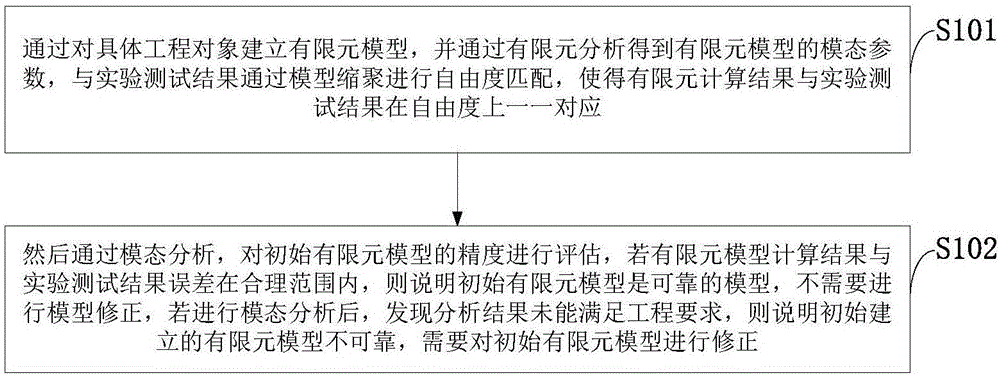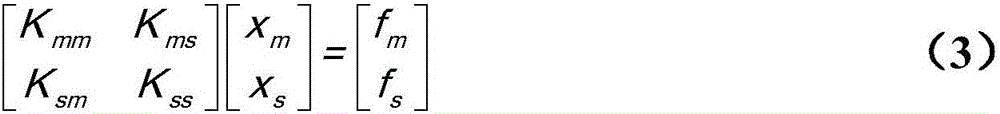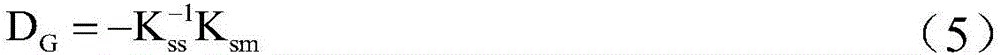# System model correction method oriented to multipoint excited vibration test

## A technology for exciting vibration and system models, applied in special data processing applications, instruments, electrical and digital data processing, etc., can solve the problems that the modal cannot be excited, increase the test cost, and limit the number of test degrees of freedom.

Inactive Publication Date: 2017-05-17
XIDIAN UNIV +1
5 Cites 12 Cited by

## AI-Extracted Technical Summary

### Problems solved by technology

There are mainly the following technical problems in the test modeling: ① The problem of modal omission. Due to the selection of the test excitation method during the test process, some modals may not be excited, resulting in modal omission and other phenomena; ② The problem of the degree of freedom of the test mode is complete , due to the function and number of sensors, the number of degrees of freedom in the test is limited, and the information of the rotational degree of freedom cannot be measu...
View more

### Method used

1) in order to facilitate finite element analysis, delete features such as chamfering, rounded corners, threaded holes that have less influence on the overall response, and part small round holes are filled to facilitate grid division and calculation;
 In the current field of model correction, the model correction method based on sensitivity analysis and the selection method of parameters to be corrected occupy a dominant position. This type of method selects the parameters to be corrected through sensitivity analysis and uses sensitivity analysis techniques to correct the parameters. Sensiti...
View more

## Abstract

The invention discloses a system model correction method oriented to a multipoint excited vibration test. The system model correction method oriented to the multipoint excited vibration test comprises the steps that Young moduli of all units are selected as parameters to be corrected, a modal reduction method is combined to perform model correction; the model correction based on sensitivity analysis combines with the modal reduction method to perform correction. The system model correction method performs model correction based on the modal reduction method, the calculation time can be greatly shortened, and the calculation efficiency is improved. A sensitivity analysis technology is introduced to finite element dynamic simulation of a multipoint excited vibration testing system, and the purpose of making a simulation result and a testing result consistent is achieved. A testing effect can be predicted through the corrected multipoint excited vibration test system model before test implementation and can directly serve as the basis and foundation of a multipoint exciting test design and control method.

Application Domain

Design optimisation/simulationSpecial data processing applications

Technology Topic

Sensitivity analysisCorrection method +12

## Image

•••## Examples

• Experimental program(1)

### Example Embodiment

 In order to make the objectives, technical solutions and advantages of the present invention clearer, the present invention will be further described in detail below in conjunction with embodiments. It should be understood that the specific embodiments described herein are only used to explain the present invention, but not to limit the present invention.
 The application principle of the present invention will be described in detail below with reference to the accompanying drawings.
 Such as figure 1 As shown, the method for system model correction for multi-point excitation vibration test provided by the embodiment of the present invention includes the following steps:
 S101: By establishing a finite element model for a specific engineering object, and obtaining the modal parameters of the finite element model through finite element analysis, matching the degree of freedom with the experimental test results through model condensation, so that the finite element calculation results and the experimental test results are in the degrees of freedom Last one-to-one correspondence;
 S102: Then evaluate the accuracy of the initial finite element model through modal analysis. If the error between the calculation result of the finite element model and the experimental test result is within a reasonable range, the initial finite element model is a reliable model and no model modification is required If the modal analysis is carried out and it is found that the analysis result fails to meet the engineering requirements, it means that the initially established finite element model is unreliable and the initial finite element model needs to be revised.
 The system model correction method for multi-point excitation vibration test provided by the embodiment of the present invention establishes a finite element model of a specific engineering object, and obtains the modal parameters of the finite element model through finite element analysis, and then passes the model with the experimental test results The polycondensation performs the degree of freedom matching, so that the finite element calculation results and the experimental test results have a one-to-one correspondence in the degrees of freedom; then through modal analysis, the accuracy of the initial finite element model is evaluated, if the finite element model calculation results are in error with the experimental test results Within a reasonable range, it means that the initial finite element model is a reliable model and does not need to be modified. If the analysis result is found to fail to meet the engineering requirements after modal analysis, it means that the initially established finite element model is unreliable and needs To revise the initial finite element model, the detailed steps are as follows:
 Step one, establishment of finite element model
 The multi-point excitation vibration test system model is divided into three parts: moving coil, fixture and aircraft. Before establishing the finite element model, it is necessary to establish a geometric model. Due to the complexity of the component model, it must be simplified as follows:
 1) In order to facilitate finite element analysis, delete features such as chamfers, rounded corners, and threaded holes that have little effect on the overall response, and fill some small round holes to facilitate mesh division and calculation;
 2) In order to ensure that the quality and center of mass of each component are consistent with the original component, the model is mass-equivalent according to the given mass and center of mass distribution table and the center of gravity coordinate formula in theoretical mechanics;
 3) Material parameters are equivalently treated according to actual properties.
 Step two, research on model condensation
 The model condensation method realizes the degree of freedom matching by reducing the system matrix scale of the finite element model. There are mainly two types of direct method and iterative method. The direct method is represented by Guyan polycondensation method and IRS polycondensation method; the iterative method has a stepwise approximation model Polycondensation method, improved Guyan-recursive reduction method, etc. The model condensation method needs to ensure that the low-order frequencies and mode shapes of the two finite element models before and after condensation are consistent.
 In the static (Guyan) reduction, it is necessary to select a set of degrees of freedom (called a set), which are the degrees of freedom reserved in the analysis. The complement of this set (called the o set) is the set of degrees of freedom to be omitted in the analysis.
 Step three, based on the sensitivity analysis model correction method
 In the current field of model correction, sensitivity analysis-based model correction methods and parameter selection methods to be corrected occupy a dominant position. This type of method selects the parameters to be corrected through sensitivity analysis and uses sensitivity analysis techniques to correct the parameters. Sensitivity analysis is based on Taylor expansion and is limited by computational efficiency and difficulty. At present, first-order sensitivity analysis and second-order sensitivity analysis dominate. Due to the truncation error of Taylor expansion, the sensitivity analysis method is an approximate method. In the actual sensitivity calculation process, the finite difference method can be introduced to approximate the first-order or second-order sensitivity to reduce the calculation amount of the derivative operation and improve the calculation efficiency.
 In the process of model modification, three elements of optimal design need to be determined: design variables, objective function and constraint conditions.
 The parameters of design variables include physical parameters and geometric parameters. In the present invention, the physical parameters of the design variables include Young's modulus of elasticity, Poisson's ratio and density; the geometric parameters of the design variables are thickness. The purpose of the present invention is that the modal calculation result of the multi-point excitation test system is consistent with the modal test result, so the objective function is the natural frequency. In order to ensure that the total mass is consistent with the actual mass of the moving parts, the constraint condition is the total mass.
 The application principle of the present invention will be further described below in conjunction with specific embodiments.
 (1) Establishment of finite element model of multi-point excitation vibration test system
 According to the principle of quality and centroid equivalence, the original model is simplified to a certain extent in Pro/E. After the model is simplified, the quality parameters of each part of the original aircraft model are provided for quality equivalence to ensure the quality and centroid of each part before and after the simplification. Consistent with the original model. Secondly, using Pro/E to analyze the quality of a certain part of the aircraft, the parameters such as volume, mass, and center of gravity can be obtained. After the simplification is completed, import the finite element software for meshing, define reasonable boundary conditions and material properties, and finally establish a finite element model.
 (2) Model condensation
 In fact, it is difficult to obtain the experimental mode shape information corresponding to all finite element nodes in the experiment. Therefore, the present invention assumes that in the simulation experiment, only the lateral degrees of freedom of some nodes can be accurately measured, and the remaining degrees of freedom are correspondingly reduced degrees of freedom. Therefore, the total number of main degrees of freedom accounts for a small part of the total number of finite element degrees of freedom, which greatly reduces the calculation time and improves the calculation efficiency.
 In order to verify the correctness of the model polycondensation, a modal analysis is carried out with an aircraft as an example. In the present invention, the Guyan reduction method is used for reduction, and the designated points are used as the set of A (nodes to be retained), and then the Lanczos method is used to solve the first three natural frequencies. The following table shows the three-order natural frequencies before and after the modal condensation.
 Table 1 Before and after modal condensation, the third-order natural frequency of the empty shaker
 Mode order Before polycondensation (HZ) After polycondensation (HZ) error Level 1 48.547 48.65 0.2％ Level 2 130.1 132.74 2％ Level 3 245.47 256.89 4.6％
 It can be seen from Table 1 that the first three-order natural frequency error before and after condensation is within 5%, so the number of degrees of freedom of the finite element model after condensation is basically the same as the number of degrees of freedom measured by the simulation experiment, but the calculation time is greatly reduced, so for large The finite element simulation of spacecraft is very necessary for model condensation.
 (3) Model correction technology based on sensitivity analysis
 For the real spacecraft structure, the density and the size of the structure are relatively easy to obtain, while the Young's modulus is difficult to obtain accurately due to the complex structure, welding, and articulation. Therefore, the present invention selects the Young's modulus and thickness of all elements as the parameters to be corrected, and performs model correction in combination with the modal polycondensation method. After the model is revised, the first few natural frequencies of the shaking table, the shaking table after installing the fixture, the aircraft, and the multi-point excitation vibration test system are shown in the following table.
 Table 2 Comparison of the first two natural frequencies of the empty shaker table after model correction and the experimental data
 Mode order Test value After model revision error Level 1 2139.5 2079.7 2.8％ Level 2 2728.5 2619.3 4％
 Table 3 Comparison of the first two natural frequencies of the shaker with the experimental data after the model is revised and the fixture is installed
 Mode order Test value After model revision error Level 1 1720.5 1652.4 3.95％ Level 2 2388.5 2491.8 4.3％
 Table 4 Comparison of the first two natural frequencies of the aircraft after model modification and the experimental data
 Mode order Test value After model revision error Level 1 51.18 49.81 2.6％ Level 2 124.75 129.9 4％
 Table 5 Comparison of the first two natural frequencies of the aircraft and the multi-point excitation vibration test system with experimental data after model modification
 Mode order Test value After model revision error Level 1 43.87 44.818 2.16％ Level 2 126.04 120.2 4.6％
 It can be seen from the above table that the first three-order natural frequency error after model modification is within 5%, which meets the target requirement. Therefore, the model is reliable and can be used for further finite element simulation analysis.
 The above are only the preferred embodiments of the present invention and are not intended to limit the present invention. Any modification, equivalent replacement and improvement made within the spirit and principle of the present invention shall be included in the protection of the present invention. Within range.

## PUM## Description & Claims & Application Information

We can also present the details of the Description, Claims and Application information to help users get a comprehensive understanding of the technical details of the patent, such as background art, summary of invention, brief description of drawings, description of embodiments, and other original content. On the other hand, users can also determine the specific scope of protection of the technology through the list of claims; as well as understand the changes in the life cycle of the technology with the presentation of the patent timeline. Login to view more.
Who we serve
• R&D Engineer
• R&D Manager
• IP Professional
Why Eureka